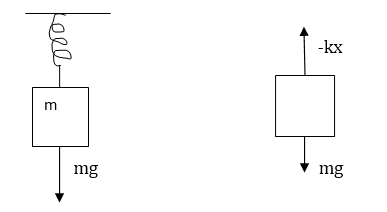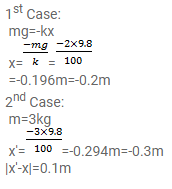# A block of 2 kg is suspended from the ceiling`
Question:

A block of $2 \mathrm{~kg}$ is suspended from the ceiling through a mass less spring of spring constant $\mathrm{k}=100 \mathrm{~N} / \mathrm{m}$. What is the elongation of the spring? If another $1 \mathrm{~kg}$ is added to the block, what would be the further elongation?

Solution: